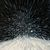# Reconstruct shape via ArcPy: How to validate geometry?

156
1
02-16-2022 08:15 AMby
Frequent Contributor

I have a field calculator Python script that I use to update the shape field of a FC.
- Densifies any true curves.
- Populates M-values of coordinates.
- Reconstructs shape.

```Expression:
-----------
new_shape( !SHAPE! )

Code Block:
-----------
def new_shape(geom):
spatial_reference = geom.spatialReference
geom = geom.densify("ANGLE", 10000, 0.174533)
parts = arcpy.Array()
for i in range(geom.partCount):
part = geom.getPart(i)
points = arcpy.Array()
for j in range(part.count):
point = part.getObject(j)
point.M = geom.measureOnLine(point)
points.append(point)
parts.append(points)
return arcpy.Polyline(parts, spatial_reference)```

Screenshot

Question:

I want to add logic to the script that will check the new geometry for issues. I know from experience that problems in the code can mean that an empty geometry gets returned to the feature (not what I want).
- I believe an empty geometry is a valid geometry. That's why the code doesn't error-out.

What kinds of things should I check for in the new geometry?
Ideas:
1. New shape isn't empty.
2. New point count vs. old point count.
3. New length vs. old length.
4. New part count vs. old part count.
5. Other?

```if new_geom   and   new_geom.pointCount>=orig_geom.pointCount   and   abs(orig_geom.length-new_geom.length) < 1   and   new_geom.partCount==orig_geom.partCount:
return new_geom
else:
return orig_geom```

Or is it unnecessary to check those things, other than checking for an empty shape, since ArcPy validates the geometry for us?

I'm using ArcMap 10.7.1. But I believe this would apply to ArcGIS Pro too. (Oracle 18c SDE.ST_GEOMETRY)byMVP Esteemed Contributor

5.  Other

Have you looked at what other things check for

Invalid Geometry—ArcGIS Pro | Documentation